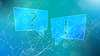# TechTopics No. 129

Interrupting capacity for system X/R ratios exceeding 17

TechTopics No. 44 discusses the shortcircuit current waveform and the basis of the high-voltage circuit breaker rating structure of IEEE Std C37.04. The ratings in the IEEE standards are based on a standard system X/R ratio of 17, which gives a time constant of decay of the dc component of the short-circuit current of 45 ms.

Siemens has been asked by customers for the interrupting capabilities of the circuit breakers for system X/R ratios exceeding 17. When the X/R is higher than 17, the time constant of dc decay becomes longer than 45 ms, so the %dc component at contact part is higher than that at X/R = 17, and the asymmetrical interrupting current is increased.

To examine this situation, consider the %dc component versus X/R ratio chart below.

This chart is a replication of the chart in IEEE Std C37.09-1999, which is figure 2, for system frequency of 60 Hz.

Unfortunately, the upcoming revision of C37.09 will not include this chart, but the underlying basis of the ratings system for the circuit breakers will not change in the new revision.

Similarly, the equations in this issue of TechTopics, which appear in the 1999 editions of IEEE Std C37.04 or C37.09 may not be included in the upcoming revisions of these standards (expected in 2017 or early 2018), even though the ratings basis (and the underlying physics) are not changing.

The bottom curve on the chart is for X/R = 17. For many Siemens circuit breakers, the minimum opening time is 25 ms, to which must be added the minimum relay operating time of 8.3 ms (one-half cycle) stipulated in the standards. This yields a contact part time of 33.3 ms, which corresponds to a rated interrupting time of 50 ms (three cycles) on a 60 Hz system.

For a contact part time of 33.3 ms, the %dc component in the graph is 47.8 percent. The exact value can be calculated using the formulas in TechTopics No. 44 (or in IEEE Std C37.09- 1999) as:

Knowing the %dc component, the asymmetrical interrupting capacity of the circuit breaker can be calculated from the following:

A simpler way to get the S-factor from the %dc component is to use the chart below:

For this example circuit breaker, this equation yields an S-factor of 1.206, and the asymmetrical interrupting capability of our example circuit breaker is 40 kA * 1.206 = 48.2 kA asymmetrical. For use on a system with an X/R ratio higher than 17, the required asymmetrical interrupting current cannot be higher than the asymmetrical current of 48.2 kA that was demonstrated during the design (type) tests to prove the interrupting capabilities of our example 40 kA symmetrical circuit breaker.

What would the equivalent symmetrical interrupting current for this circuit breaker be when applied on a system with an X/R ratio higher than 17?

For discussion, assume an X/R ratio of 30. From the equations for %dc from X/R ratio, with contact part time of 33.3 ms, the required %dc would be 65.8 percent. From the equation of S-factor from %dc, the required S-factor would be 1.366. The asymmetrical interrupting capacity demonstrated during type tests was 48.2 kA, so the equivalent symmetrical interrupting capability would be 48.2 kA/1.366 = 35.3 kA.

Consider another example, with X/R = 23. From the equations, the %dc would be 57.9 percent and the required S-factor would be 1.292, so the equivalent symmetrical interrupting capability would be 48.2 kA/1.292 = 37.3 kA.

A final word – of course, the symmetrical interrupting capability does not decrease because of the increase in X/R ratio, but the “equivalent symmetrical interrupting capability” calculated in accordance with this method is the maximum at which the circuit breaker can be applied with the higher X/R ratio, as a system with that X/R ratio and that available symmetrical current (e.g., X/R = 23, symmetrical current 37.3 kA) would be capable of producing 48.2 kA asymmetrical fault current, the maximum demonstrated during type tests with the required performance on the basis of the the standard X/R = 17. Since Siemens has no control over the short-circuit conditions, Siemens cannot guarantee that faults will always be symmetrical, and have to apply the circuit breaker for the worst case asymmetrical fault condition.